# 浅谈算法和数据结构十二 无向图相关算法基础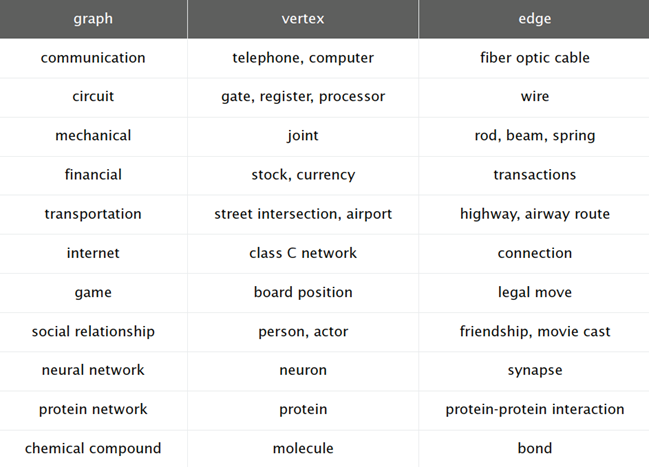# 无向图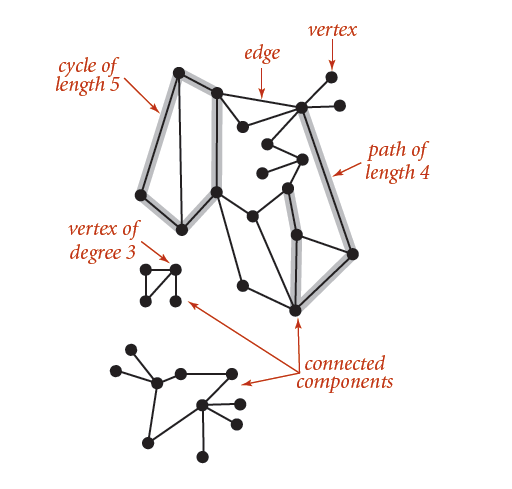# 图的API 表示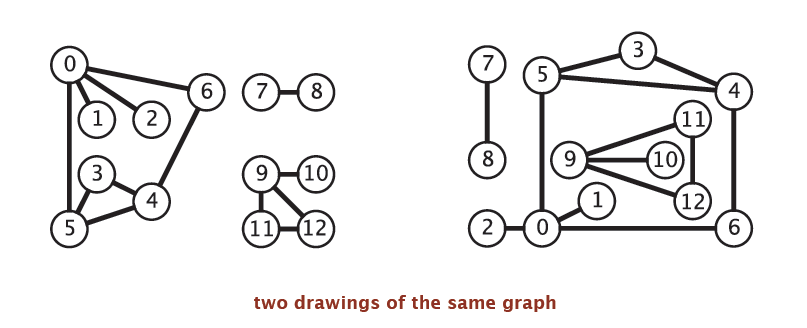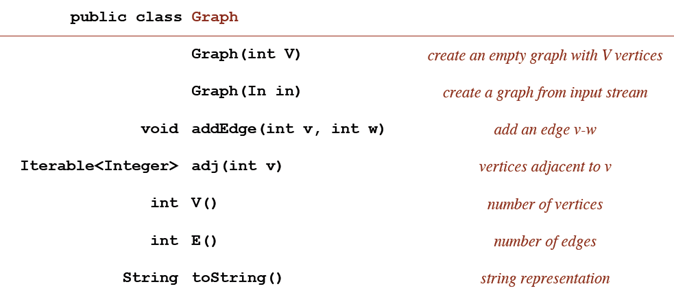Graph的API的实现可以由多种不同的数据结构来表示，最基本的是维护一系列边的集合，如下：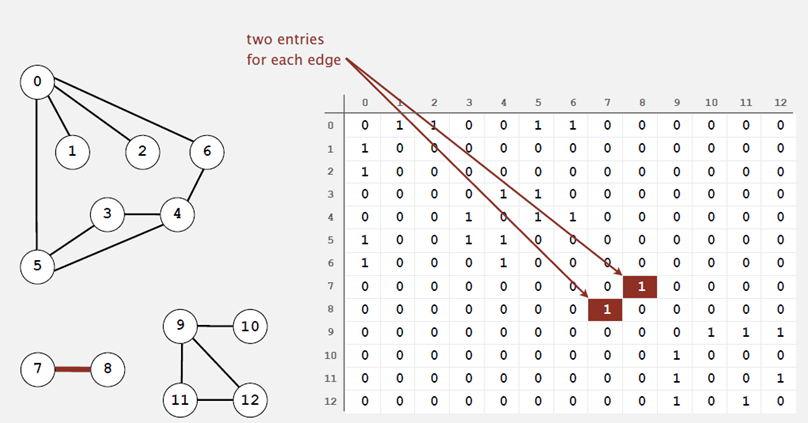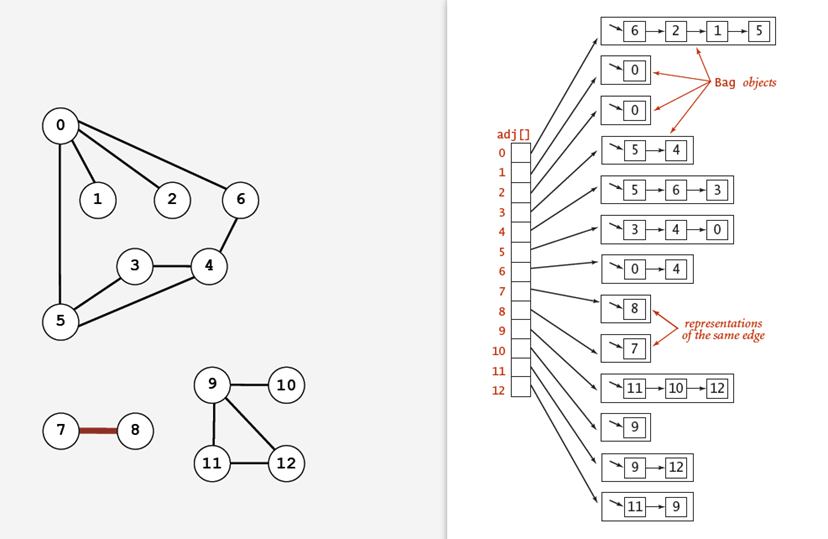``````public class Graph
{
private int edges;//边的个数

public Graph(int vertical)
{
this.verticals = vertical;
this.edges = 0;
for (int v = 0; v < vertical; v++)
{
}
}

public int GetVerticals ()
{
return verticals;
}

public int GetEdges()
{
return edges;
}

public void AddEdge(int verticalStart, int verticalEnd)
{
edges++;
}

{
}
}``````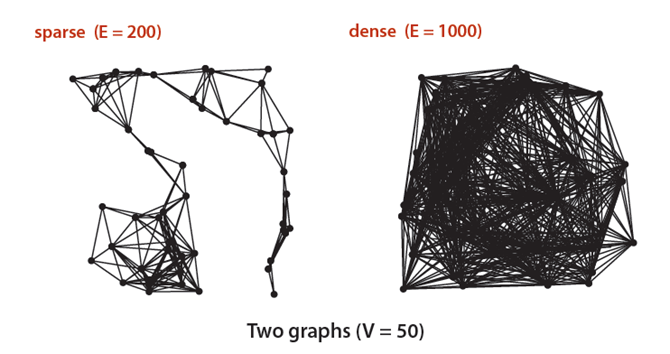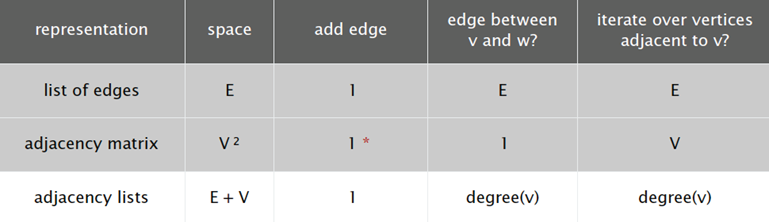# 深度优先算法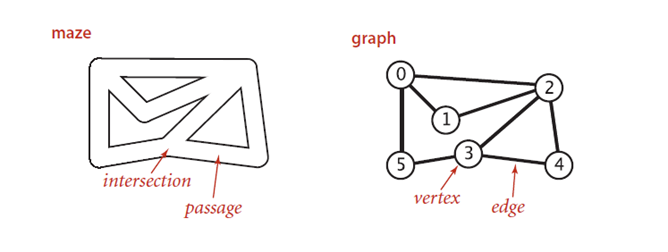• 在身后放一个绳子
• 访问到的每一个地方放一个绳索标记访问到的交会点和通道
• 当遇到已经访问过的地方，沿着绳索回退到之前没有访问过的地方：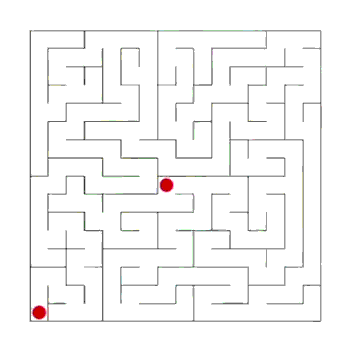• 创建一个Graph对象
• 将Graph对象传给图算法处理对象，如一个Paths对象
• 然后查询处理后的结果来获取信息

``````public class DepthFirstSearch
{
private bool[] marked;//记录顶点是否被标记
private int count;//记录查找次数

private DepthFirstSearch(Graph g, int v)
{
marked = new bool[g.GetVerticals()];
dfs(g, v);
}

private void dfs(Graph g, int v)
{
marked[v] = true;
count++;
{
if (!marked[vertical])
dfs(g,vertical);
}
}

public bool IsMarked(int vertical)
{
return marked[vertical];
}

public int Count()
{
return count;
}
}``````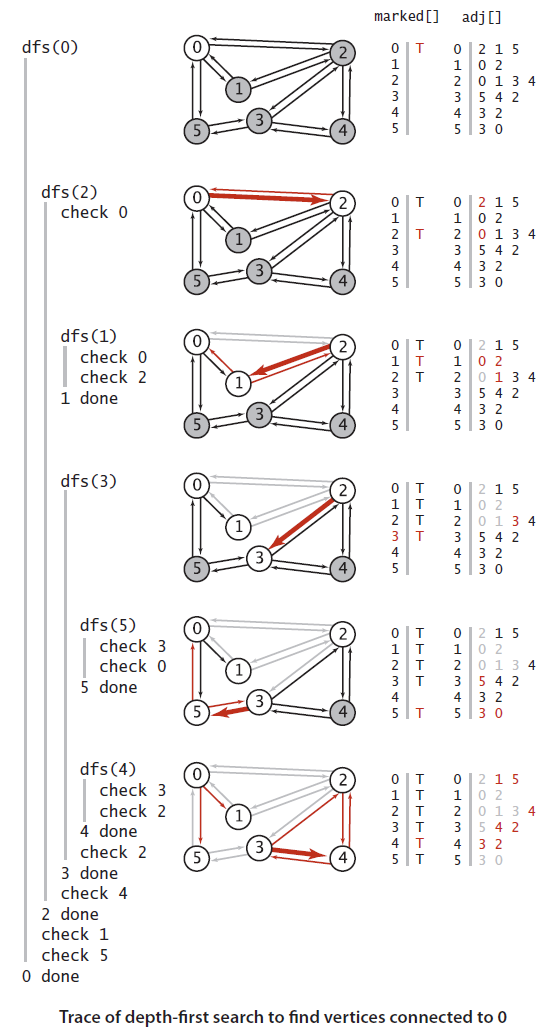# 深度优先路径查询

``````public class DepthFirstPaths
{
private bool[] marked;//记录是否被dfs访问过
private int[] edgesTo;//记录最后一个到当前节点的顶点
private int s;//搜索的起始点

public DepthFirstPaths(Graph g, int s)
{
marked = new bool[g.GetVerticals()];
edgesTo = new int[g.GetVerticals()];
this.s = s;
dfs(g, s);
}

private void dfs(Graph g, int v)
{
marked[v] = true;
{
if (!marked[w])
{
edgesTo[w] = v;
dfs(g,w);
}
}
}

public bool HasPathTo(int v)
{
return marked[v];
}

public Stack<int> PathTo(int v)
{

if (!HasPathTo(v)) return null;
Stack<int> path = new Stack<int>();

for (int x = v; x!=s; x=edgesTo[x])
{
path.Push(x);
}
path.Push(s);
return path;
}
}``````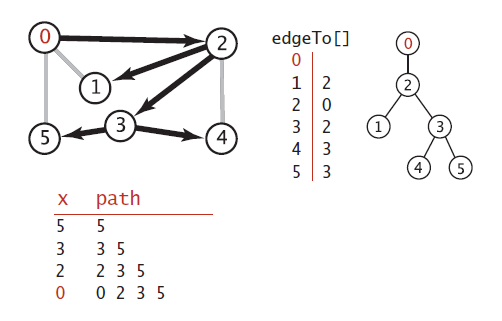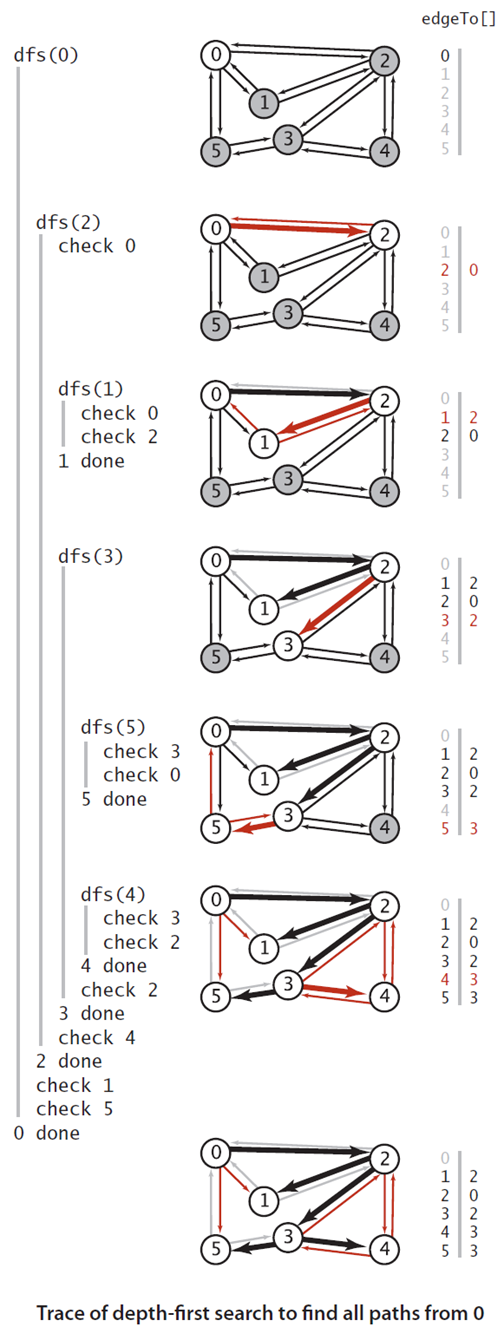# 广度优先算法

• 将 s放到FIFO中，并且将s标记为已访问
• 重复直到队列为空
1. 移除最近最近添加的顶点v
2. 将v未被访问的节点添加到队列中
3. 标记他们为已经访问

``````class BreadthFirstSearch
{
private bool[] marked;
private int[] edgeTo;
private int sourceVetical;//Source vertical

{
marked=new bool[g.GetVerticals()];
edgeTo=new int[g.GetVerticals()];
this.sourceVetical = s;
bfs(g, s);
}

private void bfs(Graph g, int s)
{
Queue<int> queue = new Queue<int>();
marked[s] = true;
queue.Enqueue(s);
while (queue.Count()!=0)
{
int v = queue.Dequeue();
{
if (!marked[w])
{
edgeTo[w] = v;
marked[w] = true;
queue.Enqueue(w);
}
}
}
}

public bool HasPathTo(int v)
{
return marked[v];
}

public Stack<int> PathTo(int v)
{
if (!HasPathTo(v)) return null;

Stack<int> path = new Stack<int>();
for (int x = v; x!=sourceVetical; x=edgeTo[x])
{
path.Push(x);
}
path.Push(sourceVetical);
return path;
}

}``````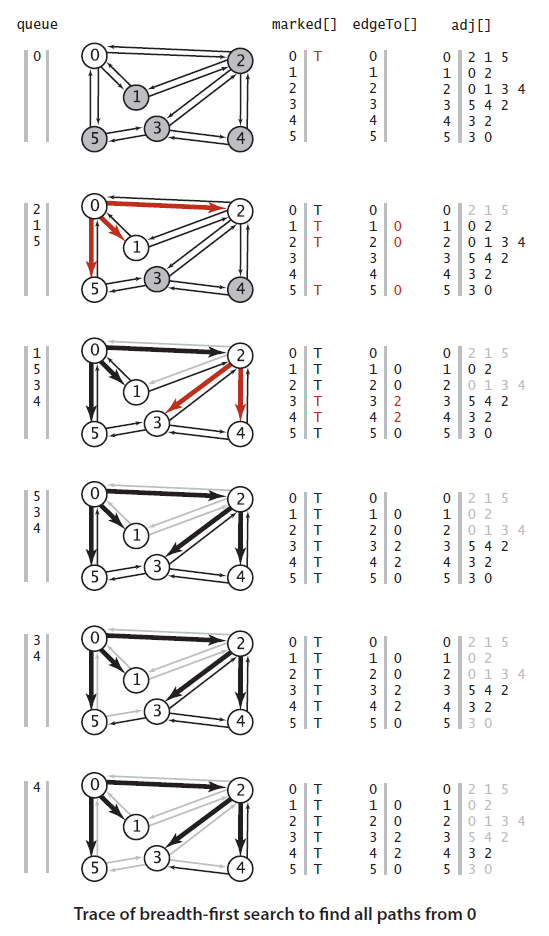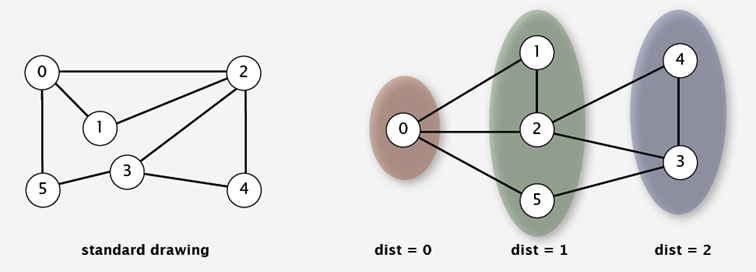# 总结

Do you have any suggestions for improvement?